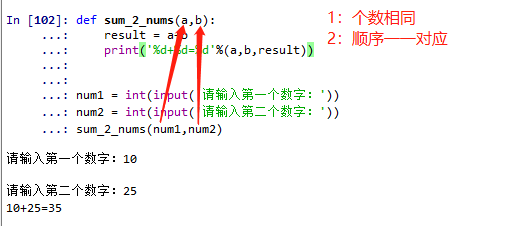• 位操作宏定义 我们在写C语言时，经常需要用到位操作，但是每次自己使用逻辑运算符进行操作的时候，很费脑子，试试下面几个宏定义吧： #define GET_BIT(value,bit) ((value)&(1<<(bit))) //读取指定位 #...
位操作宏定义
我们在写C语言时，经常需要用到位操作，但是每次自己使用逻辑运算符进行操作的时候，很费脑子，试试下面几个宏定义吧：
#define GET_BIT(value,bit) ((value)&(1<<(bit)))    //读取指定位
#define CPL_BIT(value,bit) ((value)^=(1<<(bit)))   //取反指定位

#define SET0_BIT(value,bit) ((value)&=~(1<<(bit))) //把某个位置0
#define SET1_BIT(value,bit) ((value)|= (1<<(bit))) //把某个位置1

位操作函数
这4个宏定义已经可以在大部分场合使用了，但是这个4个宏定义貌似功能也没事很全。 比如要操作寄存器的某些位，但是又不改变其他位怎么办呢，试试如下的函数吧：
//设置数据的某些位的值
/*
value 需要设置的数据的指针
bitl 需要设置的位的低位
bith 需要设置的位的高位
data 需要设置的数据
*/

void SET_DATA(unsigned int *value,unsigned int bitl,unsigned int bith,unsigned int data)
{
unsigned int* v = value;
if(bitl<=bith)
{
unsigned int bcount = bith-bitl+1;
unsigned int cbit=0;
unsigned int cdata=0;
for(unsigned int i=0;i<bcount;i++)
{
cdata |=(1<<i);
cbit  |=(1<<(bitl+i));
}
(*v)  &=~(cbit);
(*v)  |=((data&cdata)<<bitl);
}
}

使用方法: 例如 变量Value 的初始值为0xFF，要设置Value 的 0 到 5位 为 2 ，那么就调用函数:
	int Value = 0xFF;
SET_DATA(&Value,0,5,2);

展开全文• python def 定义函数，调用函数 def sum_2_nums(a,b): #def 定义函数 result = a+b print('%d+%d=%d'%(a,b,result)) num1 = int(input('请输入第一个数字：')) num2 = int(input('请输入第二个数字：')) sum_2_...
#每天一点点# python def 定义函数，调用函数
def sum_2_nums(a,b):  #def 定义函数
result = a+b
print('%d+%d=%d'%(a,b,result))

num1 = int(input('请输入第一个数字：'))
num2 = int(input('请输入第二个数字：'))
sum_2_nums(num1,num2)   #调用函数

定义和调用的函数，1：个数相同 ；2：顺序 一 一对应 这样才能正确实现展开全文调用 python
• ## 函数 def定义函数

千次阅读 2019-04-07 22:21:33
函数 def定义函数
必须参数
关于不定长参数的位置：*args放在左边，**kwargs参数放在右边
没有名字的值传给元组，有名字的传到字典，有不定长参数的默认参数放左边，没有放右边
返回值：
#返回值
# def f():
#     print('ok')
#     return 10 #作用：1 结束函数 ，2 返回一个对像
# a=f()
# print(a)
#                         #2.如果return多个对象，那么python会把多个对象封装成一个元组返回
#     Sum=0
#     for i in args:
#         Sum+=i
#     print(Sum)
#     return Sum
# print(a)

#作用域：built_in 内置作用域----global全局作用域---enclosin嵌套作用域--local本地作用域
#只有模块、类、及函数才能引入新作用域
#内部作用域要修改外部作用域变量的值时，全局变量要使用global关键字，嵌套作用域变量要使用nonlocal关键字
#nonlocal是python3新增的关键字
# count = 10
# def outer():
#     print(count)
# outer()

#递归函数:1.调用自身函数，2.有一个结束条件，3.但凡是递归可以写的循环都可以实现，效率不高
# def f(n):
#     sum_f = 1
#     for i in range(1,n) :
#         sum_f = sum_f*i
#         return sum_f
# print(f(7))
#
# def fac (n):
#     if n==1:
#         return 1
#     return n*fac(n-1)
# print(fac(9))
#斐波那契# 数列
# def fibo(n):
#   if n==0 or n==1:
#       return n
#   return fibo(n-1)+fibo(n-2)
# print(fibo(9))
#重要内置函数
# filter#过虑器
# str=['a','b','c','d']
# def fun1(s):
#     if s!='a':#过虑‘a’
#         return s
# ret = filter(fun1,str)
# print(ret)#<filter object at 0x0000000001051C88>ret是一个迭代器对象
# print(list(ret))#['b', 'c', 'd']

# map#修改器
# str=['a','b','c','d']
# def fun2(s):
#     return s +"alvin"#['aalvin', 'balvin', 'calvin', 'dalvin']
# ret = map(fun2,str)
# print(ret)#map objectr 迭代器
# print(list(ret))

# #reduce #叠加器
# from functools import reduce
#
#     return x+y

#lambda 匿名函数
from functools import reduce
print(reduce(lambda a,b :a*b,range(1,6)))
展开全文• ## 宏定义函数

千次阅读 2020-08-29 18:56:39
概念 在预编译阶段完成代码替换。 优势 (1)增加代码的可读性： 宏定义的优点很多，但我个人理解宏定义最明显的优点...宏定义函数的参数没有数据类型，因此可轻松实现模板函数的功能 #define MAX(a, b) (a > b ? a
概念
在预编译阶段完成代码替换。
优势
(1)增加代码的可读性：
宏定义的优点很多，但我个人理解宏定义最明显的优点就是增加代码的可读性。求圆的面积， 用“PI * r* r”比 “3.14人r”的可读性就强很多了。
#define PI 3.14

float circleArea(float r) {
return PI * r * r;
}

(2)自带“模版函数”的buff:
宏定义函数的参数没有数据类型，因此可轻松实现模板函数的功能
#define MAX(a, b) (a > b ? a : b)

std::cout << "max of a and b is: " << MAX(1, 2)  << std::endl;
std::cout << "max of a and b is: " << MAX(1.5, 2.1) << std::endl;

(3)相比于正常函数，宏定义函数性能更快
宏定义的本质决定了这个优势，宏定义只是在代码编译阶段做一个简单的替换，没有任何语法和优化的空间，因此宏定义函数没有正常函数所需的开销，包括：开辟空间，记录返回地址，形参压栈，从函数中返回释放堆栈。
缺点#define SUM(a,b) a+b
1 宏定义函数只能完成一些简单的替换，因此他的使用场景是有限制的，即使是宏定义函数， 也只是完成简单的功能， 不能真正的替代函数。2 由于只是做一个替换，因此使用的时候需要谨慎，避免出现符号优先级而出现的低级错误。
#define SUM(a,b) a+b
std::cout << "area of rectangle: " << 2 * SUM(1, 2) << std::endl;
// 打印的结果是4， 而不是6.因此替换后的代码：
2 * 1 + 2， 而不是2 * （ 1+ 2）

一个惯用的用法
用do{}while(0)包裹宏定义函数中的代码，这样的好处就是方便实现很复杂的宏定义函数，还可以把这个宏定义函数伪装成一个正常的函数去执行。 如下例：
#define UNIT_AREA(shape)               \
do {                                 \
if (shape == "circle") {           \
std::cout << PI << std::endl;    \
} else if ( shape == "square") {   \
std::cout << "1" << std::endl;   \
} else {                           \
std::cout << "1.0" << std::endl; \
}                                  \
} while(0)

std::cout << "area of unit area: " << std::endl;
UNIT_AREA("circle");

宏定义函数中的特殊符号
(1) …和__VA__ARGS__
支持宏定义就函数中的可变参数，宏定义参数可变，或者部分参数可变。 使用“…”配合“VA_ARGS”。
#define  LOG(...) {printf("%s\n", __VA_ARGS__);}
#define  ERROR_LOG(x, ...) if (x) {printf("%s\n", __VA_ARGS__);}

// 以下代码皆可执行
LOG("this is log")
LOG("this is log");
ERROR_LOG(1, "this is error log");
{printf("log");}
{printf("log");};

连接符：##
待写
展开全文• 定义函数： 预处理阶段进行简单的文本替换，没有入栈、出栈、参数传递和函数返回等等工作，执行效率明显高于普通函数，因此，简短并且被频繁调用的函数经常用宏定义函数来代替实现 没有参数检查，会影响程序安全 ...
• ## Mathematica定义函数

万次阅读 2015-10-30 10:05:52
Mathematica定义函数时有点特殊，需在变量后加一个下划线，具体见下面示例：
• 生成傅里叶级数分段定义函数的代码 生成定义为片段的傅立叶函数系列，生成系数A0，An，Bn和逼近函数，生成原始函数和逼近函数的图的代码。 代码获取
• 一直不明白这样写具体有什么意义。直到我准备实现一个类似与 java 中的 Timer#schedule...而要实现这种重复定时器，似乎需要使用 “函数中定义函数的”的方式才可以。 def scheduler_build(delay, cmd): def b
• 函数实质上是一个类似于单独的逻辑单元的JavaScript代码，使用函数可以使代码更为简洁，提供重用性，在JavaScript中，大约有95以上的代码是包含在函数中的，由此可见，函数在JavaScript中地位...定义函数的基本语...
• 函数内部定义函数要在函数内部调用 def paint(): print(&amp;amp;amp;quot;paint&amp;amp;amp;quot;) def paintA(): print(&amp;amp;amp;quot;paintA&amp;amp;amp;quot;) paintA() paint() ...Python
• 定义函数有两种方法： （1）函数表达式方式 let myFun=function(){ console.log("当前是函数表达式")； } （2）函数声明形式 function myFun(){ console.log("当前是函数表达式")； } 两种定义函数的...javascript
• 简介 关于函数指针的介绍，这里就不做过多说明了，不了解的同学可以...使用 typedef 定义函数指针 通过下面这个例子，我们看看 typedef 是怎么搭配函数指针使用的吧。 int add(int a, int b) { return a + b; ...typedef
• #define MAX(a,b) ((a)>(b)?(a):(b)) int MAX1(int a,int b) { return a>b?a:b; ...1）宏定义函数没有参数类型也不做类型检查，预编译...2）宏定义函数时一定要注意括号的存在和匹配，有时会因为 括号的不存在就会导致
• Python实现0-n累加求和一、使用三种方法实现0-n累加求和1、使用while循环2、使用 for循环3、使用递归函数二、使用了三种实现累加求和的方法，分别定义了三个函数...定义函数分别使用while循环、for循环、递归函数实...python 数据分析
• ## 利用typedef定义函数指针

万次阅读 多人点赞 2018-06-20 16:14:13
利用typedef定义函数指针2015年08月18日 10:56:46阅读数：4151利用typedef定义函数指针进入正文：　代码简化, 促进跨平台开发的目的.　typedef 行为有点像 #define 宏，用其实际类型替代同义字。　不同点：typedef ...
• 定义函数 定义函数就是封装一部分代码，设置参数，输入相应的参数就会返回相应的结果。如果一个爬虫的代码超过五十行，那么如果不定义函数，每一行代码就会看起来很凌乱，如果定义一个函数的话，界面的结构就会很...爬虫 python
• 函数类型 又叫 函数签名 ， 一个函数 的类型就是函数定义首行去掉函数名、参数名和{，可以 使用fmt.Printf 的”%T”格式化参数打印函数的类型。 函数类型相同 两个函数类型相同的条件是:拥有相同的形参列表和返回值...
• ## matlab如何在文中定义函数

万次阅读 多人点赞 2017-01-04 14:12:54
matlab如何在文中定义函数 通常我们多是将函数单独在编写在单个的m文件中，再于主程序中调用。但是，有时候觉得程序太小，直接在程序末尾编写函数，又会收到“此上下文中不允许函数定义”的错误提示。那么，matlab...matlab 脚本
• 一、typedef定义函数 除了函数指针，普通函数也可以用typedef,如：  typedef int F (void);//定义函数类型F  之后可以这样声明：  F f,g;  相当于声明  int f(void);  int g(void); 下面这个函数声明...typedef
• 结构体内不能有函数的代码， 但可以有函数的指针 c++可以直接定义函数
• ## C++struct里定义函数

万次阅读 2016-09-28 16:31:56
c++中允许在结构体当中定义函数，它的用法和类的用法很像，不过与类有一个区别在于，struct中定义的函数和变量都是默认为public的，但class中的则是默认为private。#include #include struct Person { Person(std:...
• ## C++宏定义函数

千次阅读 2019-04-13 15:45:48
这句几乎每个类都有，一开始以为是TypeName（“xxx”）调用构造函数，细看不太对，应该是调用宏定义函数，这个宏函数原型在\$SRC/OpenFOAM/db/typeInfo文件里定义的。 如下： #define TypeName(TypeNameString) ...C++
• JavaScript 使用关键字 function 定义函数 函数可以通过声明定义，也可以是一个表达式。 函数声明 在之前的教程中，你已经了解了函数声明的语法 : ? 1 2 3 function ...javascript
• Qt中快速定义函数的小技巧 1.简介 在Qt creator中编写函数的时候，在头文件编写了函数，需要在相应的cpp文件中编写对应的函数定义实现，如果每次都需要手动的敲击全部的代码，这会非常的耗时耗力，显得很方便，这...qt5
• 修饰符 返回的类型 函数名（参数类型 形式参数1,参数类型 形式参数2,......）{ 执行语句; return 返回值;}特殊情况： 功能没有具体的返回值;... 定义函数时，函数的结果应该返回给调用者，交由调用者处...java
• ## def定义函数

千次阅读 2019-03-19 19:12:16...